#Data Structure_堆_二叉树_并查集

0
0
01. 云栖社区>
2. 博客>
3. 正文

# 堆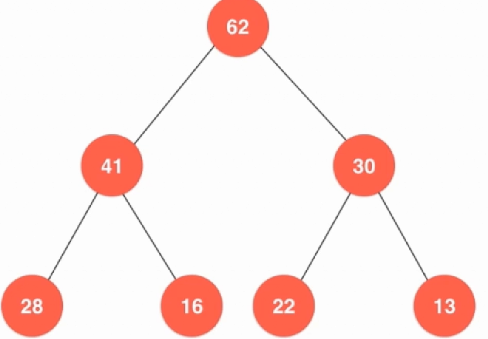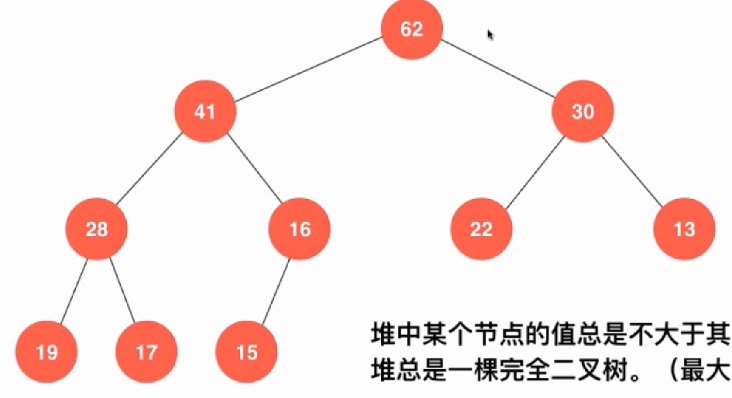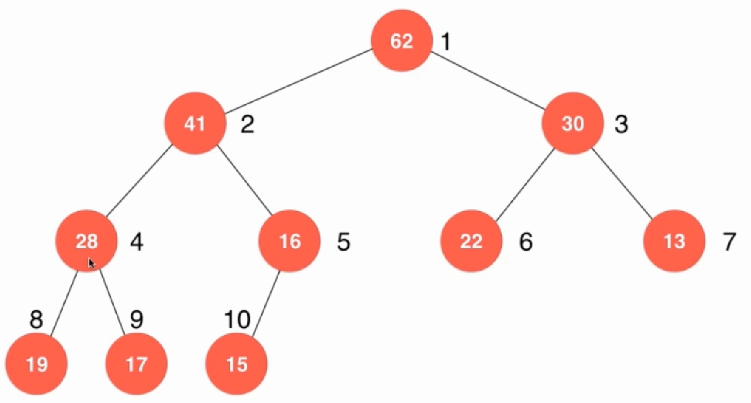``````template<typename item>
class MaxHeap {
private:
item *data;
int count = 0;
public:
MaxHeap(int capacity){
data = new item[capacity + 1];
count = 0;
}
~MaxHeap(){
delete[] data;
}
int size(){
return count;
}
bool isEmpty(){
return count == 0;
}
};

``````

``````    void shiftUp(int index) {
while (index > 1 && data[index / 2] < data[index]) {
swap(data[index / 2], data[index]);
index /= 2;
}
}
void insert(item number) {
assert(count + 1 <= capacity);
data[count + 1] = number;
count++;
shiftUp(count);
}
``````

``````    void shiftDown(int index) {
while (2 * index <= count) {
int change = 2 * index;
if (change + 1 < count && data[change + 1] > data[change]) {
change++;
}
if (data[change] <= data[index]) {
break;
}
swap(data[change], data[index]);
index = change;
}
}
item pop() {
assert(count > 0);
item target = data;
swap(data, data[count]);
count--;
shiftDown(1);
return target;
}

``````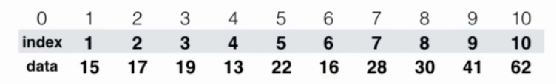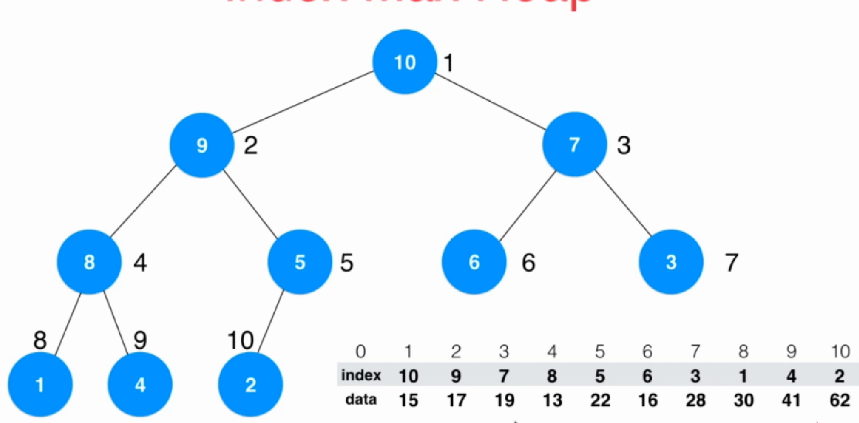``````template<typename item>
class IndexHeap {
private:
item *indexes;
item *data;
int count;
int capacity;
``````

shiftDown和shiftUp操作其实就是变一下，把交换的对象换成索引即可：

``````    void shiftUp(int k) {
while (k > 1 && data[indexes[k / 2]] < data[indexes[k]]) {
swap(indexes[k / 2], indexes[k]);
k /= 2;
}
}

void shitDown(int k) {
while (2 * k <= count) {
int change = 2 * k;
if (change + 1 <= count && data[indexes[change]] < data[indexes[change + 1]]) {
change++;
}
if (data[indexes[change]] <= data[indexes[k]]) {
break;
}
swap(indexes[change], indexes[k]);
k = change;
}
}
``````

``````    void insert(int i, item itemNumber) {
assert(count + 1 <= capacity);
assert(i + 1 >= 1 && i + 1 < capacity);
i++;
data[i] = itemNumber;
indexes[++count] = i;
shiftUp(count);
}

item extractMax(){
item num = data[indexes];
swap(indexes, indexes[count]);
count -- ;
shitDown(1);
return num;
}

``````

``````    void change(int i, item itemNumber){
i += 1;
data[i] = itemNumber;
for (int j = 1; j <= count; ++j) {
if (indexes[j] == i){
shiftUp(j);
shitDown(j);
return;
}
}
}
``````

``````template<typename Item>
class IndexMinHeap{

private:
Item *data;
int *indexes;
int *reverse;

int count;
int capacity;

void shiftUp( int k ){

while( k > 1 && data[indexes[k/2]] > data[indexes[k]] ){
swap( indexes[k/2] , indexes[k] );
reverse[indexes[k/2]] = k/2;
reverse[indexes[k]] = k;
k /= 2;
}
}

void shiftDown( int k ){

while( 2*k <= count ){
int j = 2*k;
if( j + 1 <= count && data[indexes[j]] > data[indexes[j+1]] )
j += 1;

if( data[indexes[k]] <= data[indexes[j]] )
break;

swap( indexes[k] , indexes[j] );
reverse[indexes[k]] = k;
reverse[indexes[j]] = j;
k = j;
}
}

public:
IndexMinHeap(int capacity){

data = new Item[capacity+1];
indexes = new int[capacity+1];
reverse = new int[capacity+1];

for( int i = 0 ; i <= capacity ; i ++ )
reverse[i] = 0;

count = 0;
this->capacity = capacity;
}

~IndexMinHeap(){
delete[] data;
delete[] indexes;
delete[] reverse;
}

int size(){
return count;
}

bool isEmpty(){
return count == 0;
}

void insert(int index, Item item){
assert( count + 1 <= capacity );
assert( index + 1 >= 1 && index + 1 <= capacity );

index += 1;
data[index] = item;
indexes[count+1] = index;
reverse[index] = count+1;
count++;
shiftUp(count);
}

Item extractMin(){
assert( count > 0 );

Item ret = data[indexes];
swap( indexes , indexes[count] );
reverse[indexes[count]] = 0;
reverse[indexes] = 1;
count--;
shiftDown(1);
return ret;
}

int extractMinIndex(){
assert( count > 0 );

int ret = indexes - 1;
swap( indexes , indexes[count] );
reverse[indexes[count]] = 0;
reverse[indexes] = 1;
count--;
shiftDown(1);
return ret;
}

Item getMin(){
assert( count > 0 );
return data[indexes];
}

int getMinIndex(){
assert( count > 0 );
return indexes-1;
}

bool contain( int index ){
return reverse[index+1] != 0;
}

Item getItem( int index ){
assert( contain(index) );
return data[index+1];
}

void change( int index , Item newItem ){

assert( contain(index) );
index += 1;
data[index] = newItem;

shiftUp( reverse[index] );
shiftDown( reverse[index] );
}

};
``````

# Tree

### 二叉树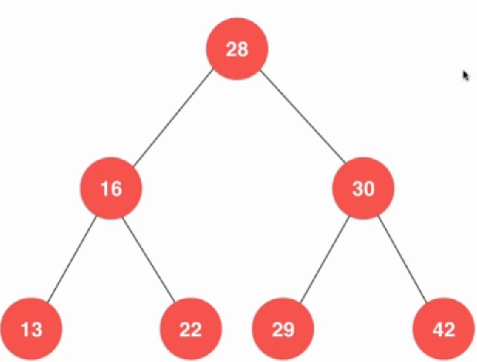``````template<typename Key, typename Value>
class BST{
private:
struct Node{
Key key;
Value value;
Node *left;
Node *right;
Node(Key key, Value value){
this->key = key;
this->value = value;
this->left = this->right = NULL;
}
};
Node *root;
int count;
public:
BST(){
root = NULL;
count = 0;
}
~BST(){
//TODO
}
int size(){
return count;
}
bool isEmpty(){
return count == 0;
}
};
``````

``````    void insert(Key key, Value value){
root = insert(root, key, value);
}

private:
Node *insert(Node *node, Key key, Value value){
if (node == NULL){
count ++;
return new Node(key, value);
}
if (node->key == key){
node->value = value;
} else if(key < node->key){
node->left = insert(node->left, key, value);
} else{
node->right = insert(node->right, key, value);
}
return node;
}

``````

``````    Value *search(Node *node, Key key){
if (NULL == node){
return NULL;
} else if(node->key == key){
return &(node->value);
} else if(key < node->key){
return search(node->left, key);
} else{
return search(node->right, key);
}
}

bool contain(Node *node, Key key){
if (NULL == node){
return false;
}
if (node->key == key){
return true;
} else if(key < node->key){
return contain(node->left, key);
} else{
return contain(node->right, key);
}
}
};
``````

``````    Value *search(Key key){
return search(root, key);
}

bool contain(Key key){
return contain(root, key);
}
``````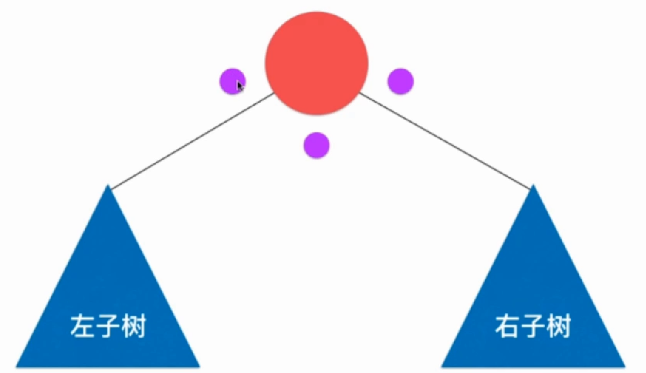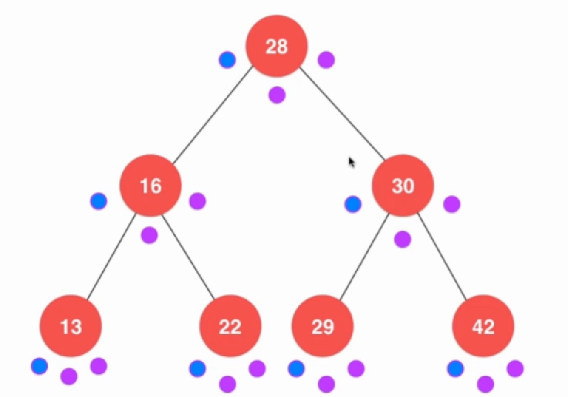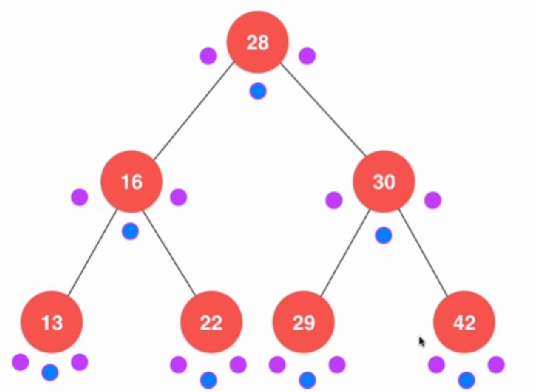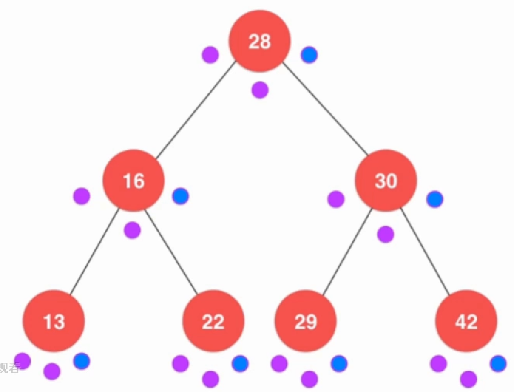``````    void preOrder(Node *node){
if (node != NULL){
cout << node->value;
preOrder(node->left);
preOrder(node->right);
}
}

void inOrder(Node *node){
if (node != NULL){
inOrder(node->left);
cout << node->value;
inOrder(node->right);
}
}

void postOrder(Node * node){
if (node != NULL){
postOrder(node->left);
postOrder(node->right);
cout << node->value;
}
}
``````

``````    void destory(Node *node){
if (node != NULL){
destory(node->left);
destory(node->right);
delete node;
count --;
}
}
``````

``````    void levelOrder(){
queue<Node *> q;
q.push(root);
while (!q.empty()){
Node *p = q.front();
q.pop();
cout << p->value;
if (p->left){
q.push(p->left);
}
if (p->right){
q.push(p->right);
}
}
}
``````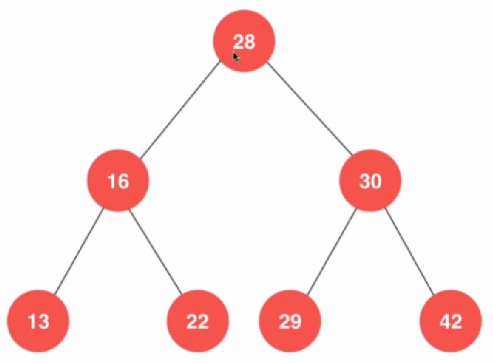``````    Key minimum() {
assert(count != 0);
Node *node = minimum(root);
return node->key;
}

Key maximum(){
assert(count != 0);
Node *node = maximum(root);
return node->key;
}
``````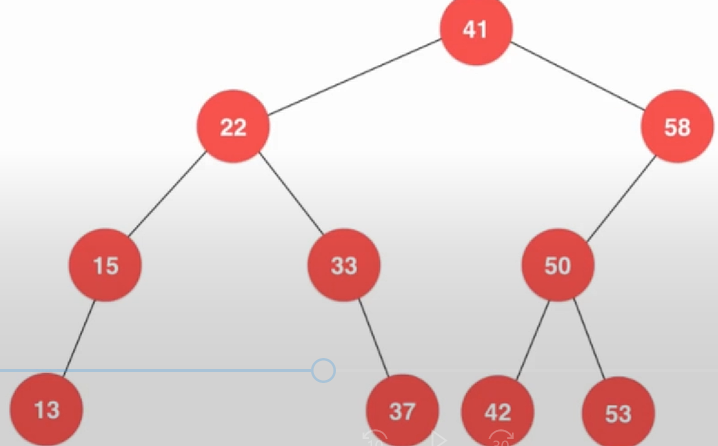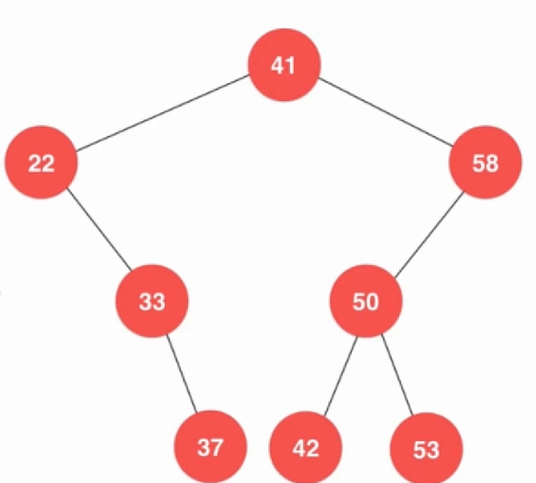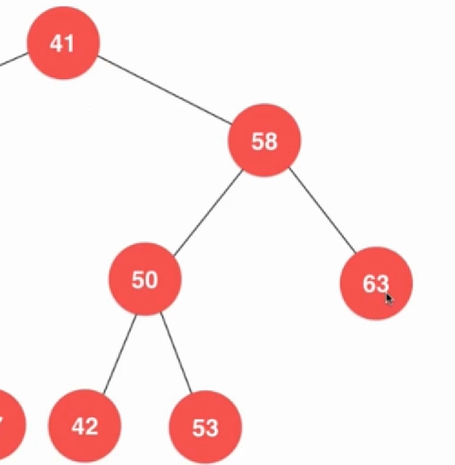``````    Node *removeMax(Node *node){
if (node->right == NULL){
Node *left = node->left;
delete node;
count --;
return left;
}
node->right = removeMax(node->right);
return node;
}

Node *removeMin(Node *node){
if (node->left == NULL){
Node *right = node->right;
delete node;
count --;
return right;
}
node->left = removeMin(node->left);
return node;
}
``````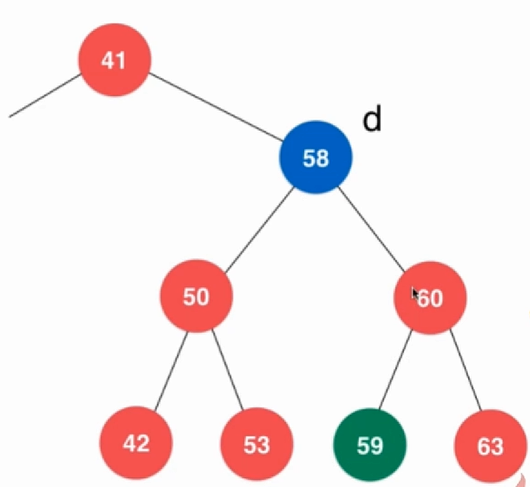，如果59没有那么就是删除60了，很明显，就是右子树的最小值了。

``````    Node *remove(Node *node, Key key){
if ( node == NULL){
return NULL;
} else if (key < node->key){
node->left = remove(node->left, key);
} else if (key > node->key){
node->right = remove(node->right, key);
} else{
if (node->left == NULL){
Node *right = node->right;
delete node;
count --;
return right;
} else if (node->right == NULL){
Node *left = node->left;
delete node;
count --;
return left;
} else{
Node *delNode = node;
Node *successor = new Node(minimum(node->right));
count ++;
successor->right = removeMin(node->right);
successor->left = node->left;
delete delNode;
count --;
return successor;
}
}
}
``````

# 并查集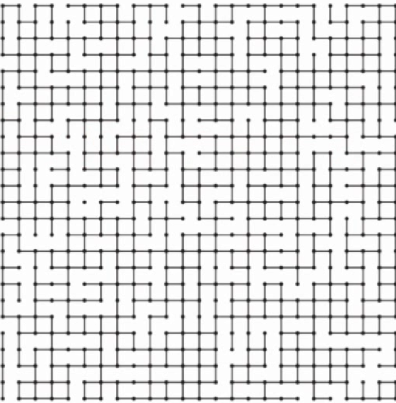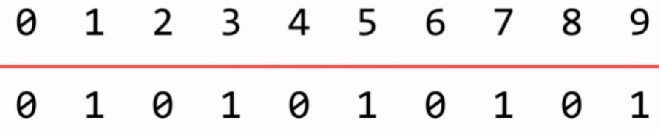``````class unionFind {
private:
int *id;
int count;
public:
unionFind(int n) {
count = n;
id = new int(n);
for (int i = 0; i < n; ++i) {
id[i] = i;
}
}

~unionFind() {
delete[] id;
}
``````

``````    int find(int p) {
assert(p >= 0 && p <= count);
return id[p];
}
``````

``````    void unionElements(int p, int q){
int pID = find(p);
int qID = find(q);
if (pID == qID){
return;
}
for (int i = 0; i < count; ++i) {
if (id[i] == pID){
id[i] = qID;
}
}
}
``````

``````    UF_version1::unionFind uF = UF_version1::unionFind(10);
uF.unionElements(1, 2);
uF.unionElements(5, 4);
uF.unionElements(3, 1);
cout << uF.isConnected(4, 5) << endl;
``````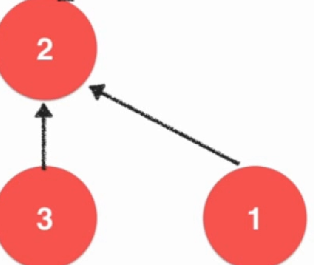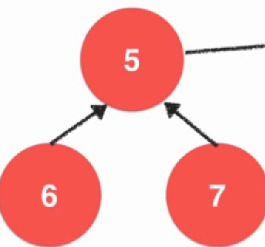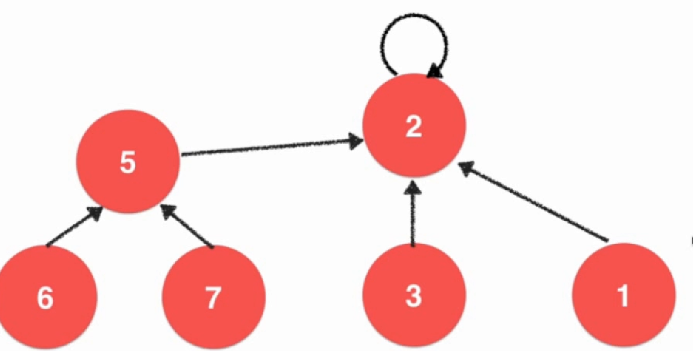``````        int find(int p){
assert(p >= 0 && p <= count);
while (parent[p] != p){
p = parent[p];
}
return p;
}

bool isConnected(int p, int q){
return find(p) == find(q);
}

void unionElements(int p, int q){
int pRoot = find(p);
int qRoot = find(q);
if (pRoot == qRoot){
return;
}
parent[pRoot] = qRoot;
}
``````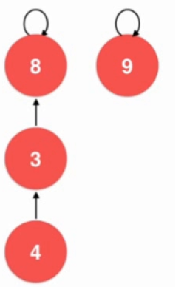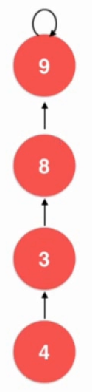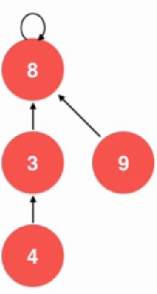``````    private:
int *parent;
int *sz;
int count;
``````

``````        void unionElements(int p, int q) {
int pRoot = find(p);
int qRoot = find(q);
if (pRoot == qRoot) {
return;
}
if (sz[pRoot] < sz[pRoot]){
parent[pRoot] = qRoot;
sz[qRoot] += sz[pRoot];
} else{
parent[qRoot] = pRoot;
sz[pRoot] += sz[qRoot];
}
}
``````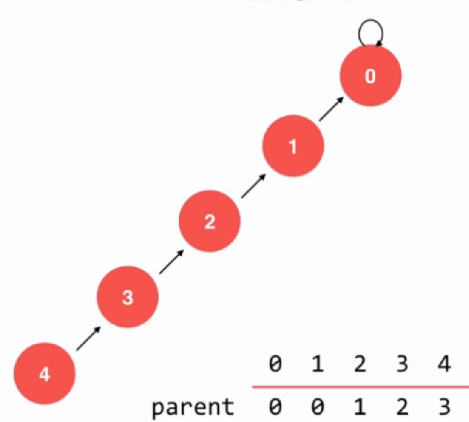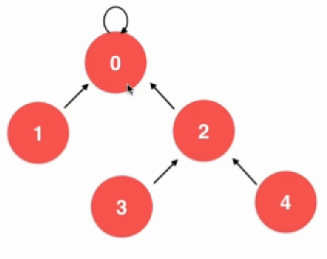``````        int find(int p) {
assert(p >= 0 && p <= count);
while (parent[p] != p) {
parent[p] = parent[parent[p]];
p = parent[p];
}
return p;
}
``````

+ 关注